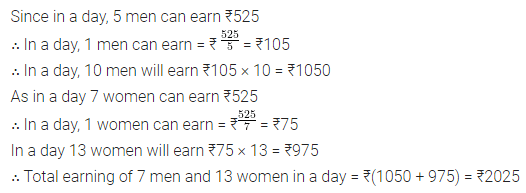# ML Aggarwal Class 8 Solutions for ICSE Maths Chapter 9 Direct and Inverse Variation Ex 9.1

## ML Aggarwal Class 8 Solutions for ICSE Maths Chapter 9 Direct and Inverse Variation Ex 9.1

Question 1.
Observe the following tables and find if x and y are directly proportional:
(i)(ii)Solution: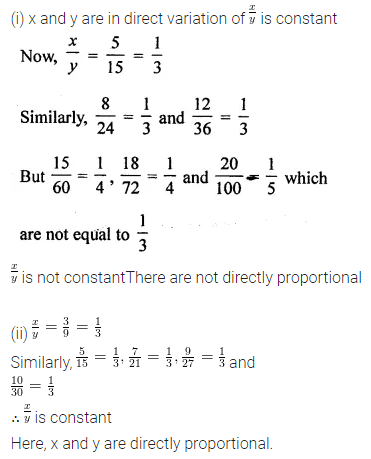Question 2.
If x and y are in direct variation, complete the following tables:
(i)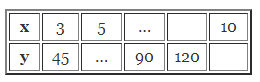(ii)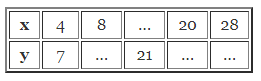Solution: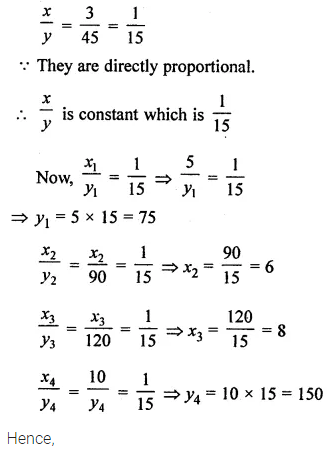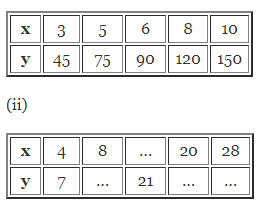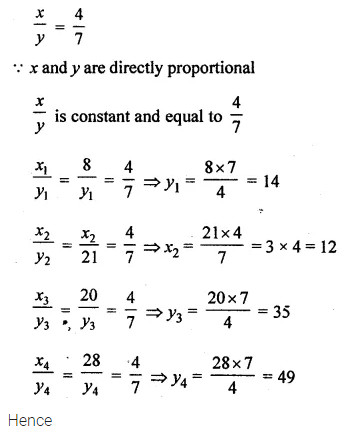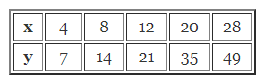Question 3.
If 8 metres cloth costs ₹250, find the cost of 5·8 metres of the same cloth.
Solution:Question 4.
If a labourer earns ₹672 per week, how much will he earn in 18 days?
Solution: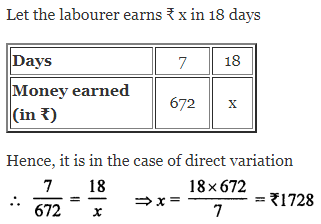Question 5.
If 175 dollars cost ₹7350, how many dollars can be purchased in ₹24024?
Solution: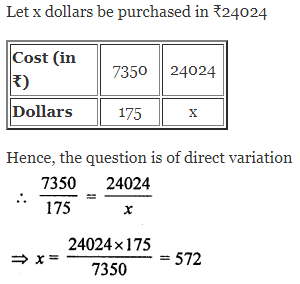Question 6.
If a car travels 67·5 km in 4·5 litres of petrol, how many kilometres will it travel in 26-4 litres of petrol?
Solution:Question 7.
If the thickness of a pile of 12 cardboard sheets is 45 mm, then how many sheets of the same cardboard would be 90 cm thick?
Solution: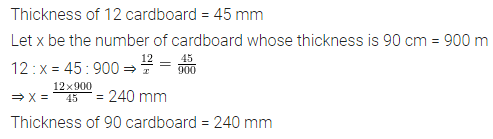Question 8.
In a model of a ship, the mast (flagstaff) is 6 cm high, while the mast of the actual ship is 9 m high. If the length of the ship is 33 m, how long is the model of the ship?
Solution: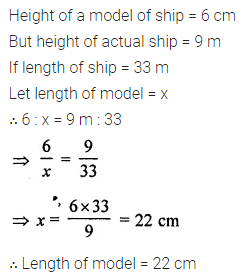Question 9.
The mass of an aluminium rod varies directly with its length. If a 16 cm long rod has a mass of 192 g, find the length of the rod whose mass is 105 g.
Solution: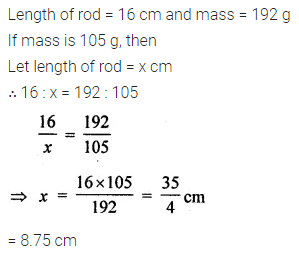Question 10.
Anita has to drive from village A to village B. She measures a distance of 3.5 cm between these villages on the map. What is the actual distance between the villages if the map scale is 1 cm = 20 km?
Solution:Question 11.
A 23 m 75 cm high water tank casts a shadow 20 m long. Find at the same time;
(i) the length of the shadow cast by a tree 9 m 50 cm high.
(ii) the height of the tree if the length of the shadow is 12 m.
Solution: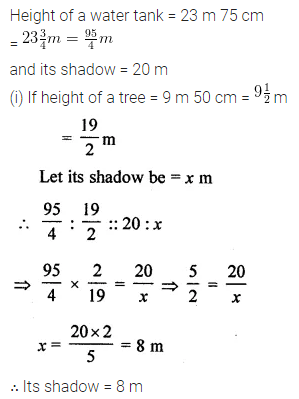Question 12.
If 5 men or 7 women can earn ₹525 per day, how much would 10 men and 13 women will earn per day.
Solution: